Math
Topics:
Line Sweep Technique

# Line Sweep Technique

Pre-requisite: Computational Geometry- I
In this article, we'll learn some algorithms based on the simple tools of computational geometry.
What is a sweep line?
A sweep line is an imaginary vertical line which is swept across the plane rightwards. That's why, the algorithms based on this concept are sometimes also called plane sweep algorithms. We sweep the line based on some events, in order to discretize the sweep.
The events are based on the problem we are considering , we'll see them in the algorithms discussed below. Other than events, we maintain a data structure which stores the events generally sorted by y coordinates (the criteria for ordering of data structure may vary sometimes) which is helpful in the processing when we encounter some event. At any instance, the data structure stores only the active events.
One other thing to note is that the efficiency of this technique depends on the data structures we use. Generally , we can use set in C++ but sometimes we require some extra information to be stored, so we go for balanced binary tree.

Let's consider our first algorithm:

Closest Pair
Problem: Find the closest pair of points in the given array of $N$ distinct points
This problem can be solved by comparing all pairs of points , but then its complexity is $O(N^2)$
So, we need a better algorithm for this . Here, we'll discuss it using line sweep technique.

For this problem, we can consider the points in the array as our events.
And in a set, we store the already visited points sorted by y coordinate.
So, first we sort the points in x direction as we want our line to move towards right.
Now, suppose we have processed the points from 1 to N-1, and let h be the shortest distance we have got so far. For Nth point, we want to find points whose distance from Nth point is less than or equal to h.
Now, we know we can only go till h distance from $x_N$ to find such point, and in the y direction we can go in h distance upwards and h distance downwards. So, all such points whose x coordinate lie in [$x_N-h,x_N$] and y coordinates lie in [$y_N-h , y_N+h$] are what we are concerned with and these form the active events of the set . All points in the set with x coordinates less than $x_N-h$ are to be deleted . After this processing, we'll add the Nth point to the set.
One thing to note is that at any instance, the number of points which are active events is O(1)(there can be atmost 5 points around a point which are active excluding the point itself).
Okay, that's a lot of theory, let's see this algo running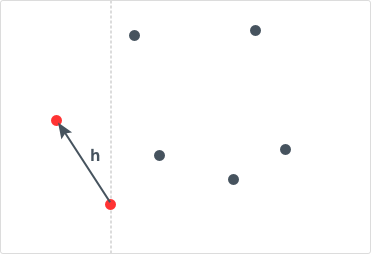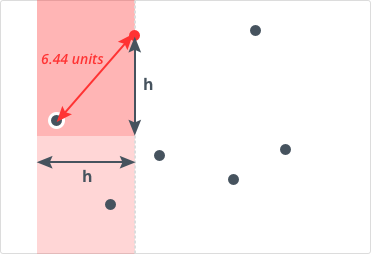Initialize h as the distance between first two points Update the value of h if present distance between points is less than h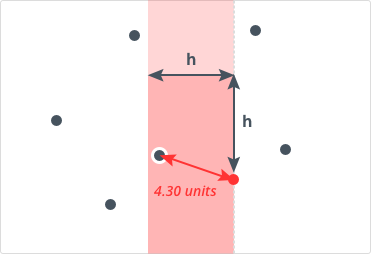The red region in the image is the region containing points which are to be evaluated with the current point.The points left to this region are removed from the set.
Following is the C++ code for above algorithm:

#define px second
#define py first
typedef pair<long long, long long> pairll;
pairll pnts [MAX];
int compare(pairll a, pairll b)
{
return a.px<b.px;
}
double closest_pair(pairll pnts[],int n)
{
sort(pnts,pnts+n,compare);
double best=INF;
set<pairll> box;
box.insert(pnts);
int left = 0;
for (int i=1;i<n;++i)
{
while (left<i && pnts[i].px-pnts[left].px > best)
box.erase(pnts[left++]);
for(typeof(box.begin()) it=box.lower_bound(make_pair(pnts[i].py-best, pnts[i].px-best));it!=box.end() && pnts[i].py+best>=it->py;it++)
best = min(best, sqrt(pow(pnts[i].py - it->py, 2.0)+pow(pnts[i].px - it->px, 2.0)));
box.insert(pnts[i]);
}
return best;
}


Let's break down the points in the code:
1. First ,we have sorted the array of points on x coordinates.
2. Then we inserted the first point in the pnts array to the set box. Note we have defined py as the first in the pair, so set will be sorted by y coordinates.
3. In the loop , for each point in pnts, we are removing the points to the left of the current point whose x coordinate has more distance than h(the current minimum distance) from $x_N$.This loop runs for overall $O(N)$ as we have only N elements in the set. The complexity of erase is $O(log N)$. So, the overall complexity of this loop is $O(N * log N)$
4. In the second for loop , we are iterating over all points whose x coordinates lie in [$x_N-h,x_N$] and y coordinates lie in [$y_N-h , y_N+h$]. Finding the lower_bound takes $O(log N)$ and this loop runs for atmost 5 times.
5. For each point, insert into the set. This step takes $O(log N)$.

So, overall time complexity of the algorithm is $O(N* log N)$
Now, let's move on to our next problem.

Union Of Rectangles:
Problem: Given a set of $N$ axis aligned rectangles(edges of rectangles parallel to x axis or y axis), find the area of union of all of the rectangles. A rectangle is represented by two points , one lower-left point and one upper-right point.
The events for this problem are the vertical edges.When we encounter a left edge, we do some action and when we encounter a right edge, we do some other action.Left edge is represented by lower-left point and right edge by upper-right point

We start our algorithm by sorting the events by x coordinates. When a lower left point of a rectangle is hit (i.e., we encounter left edge of rectangle), we insert the rectangle into the set . When we hit an upper right point of a rectangle (we encounter right edge of rectangle), we remove the rectangle from the set. At any instance, the set contains only the rectangles which intersect the sweep line (rectangles whose left edges are visited but right edges are not).

The area swept at any instance is = $\Delta$y * $\Delta$x where $\Delta$y is the length of the sweep line which is actually cut by the rectangle(s) (sum of the vertical lengths of the orange region, in the figure below) and $\Delta$x is the distance between two events of this sweep line.
But here we just know which are the rectangles intersecting the sweep line. So, here we have a new problem: how to find the length of the sweep line cut by the rectangles?
The solution to this problem is pretty the same we have been doing by now. We use the line sweep technique to find this but this time we apply it 90 degrees rotated, i.e., we sweep a horizontal line from bottom to up . The events for this sweep line would be the horizontal edges of the active rectangles(rectangles cut by vertical sweep line). When we encounter a bottom horizontal edge of an active rectangle, we increment the counter (counter here maintains the number of rectangles that overlap at current time) and we decrement it on top horizontal edge of active rectangle. When the counter becomes zero from some non zero value, we have found cut length of the vertical sweep line, so we add the area to our final answer.
Let's see it running now: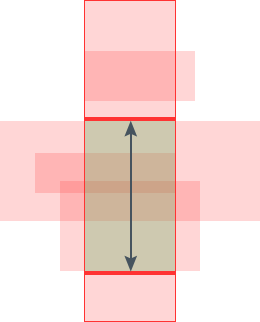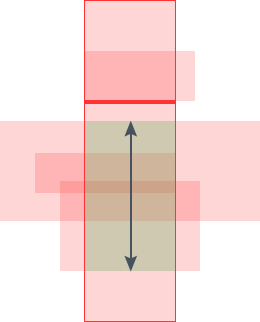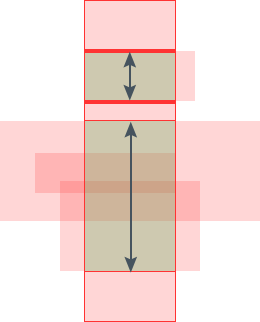The images above show how we're doing the horizontal sweep bottom up. $\Delta$y is the sum of the length of the two arrows shown in the last image. This we do, for all events of vertical sweep line.
This was our algorithm. So, let's come to implementation part. For every event of vertical sweep line , we need to find the length of the cut on the sweep line, that means we need to go for horizontal sweep line. Here, we may use boolean array as our data structure because we would have once sorted the rectangles in order of vertical edges(vertical sweep) and once in order of horizontal edges(horizontal sweep), so we would have the sorting in both the directions.
Following is the C++ code for the algorithm:

#define MAX 1000
struct event
{
int ind; // Index of rectangle in rects
bool type; // Type of event: 0 = Lower-left ; 1 = Upper-right
event() {};
event(int ind, int type) : ind(ind), type(type) {};
};
struct point
{
int x, y;
};
point rects [MAX]; // Each rectangle consists of 2 points:  = lower-left ;  = upper-right
bool compare_x(event a, event b) { return rects[a.ind][a.type].x<rects[b.ind][b.type].x; }
bool compare_y(event a, event b) { return rects[a.ind][a.type].y<rects[b.ind][b.type].y; }
int union_area(event events_v[],event events_h[],int n,int e)
{
//n is the number of rectangles, e=2*n , e is the number of points (each rectangle has two points as described in declaration of rects)
bool in_set[MAX]={0};int area=0;
sort(events_v, events_v+e, compare_x);  //Pre-sort of vertical edges
sort(events_h, events_h+e, compare_y); // Pre-sort set of horizontal edges
in_set[events_v.ind] = 1;
for (int i=1;i<e;++i)
{ // Vertical sweep line
event c = events_v[i];
int cnt = 0; // Counter to indicate how many rectangles are currently overlapping
// Delta_x: Distance between current sweep line and previous sweep line
int delta_x = rects[c.ind][c.type].x - rects[events_v[i-1].ind][events_v[i-1].type].x;
int begin_y;
if (delta_x==0){
in_set[c.ind] = (c.type==0);
continue;
}
for (int j=0;j<e;++j)
if (in_set[events_h[j].ind]==1)                 //Horizontal sweep line for active rectangle
{
if (events_h[j].type==0)                //If it is a bottom edge of rectangle
{
if (cnt==0) begin_y = rects[events_h[j].ind].y; // Block starts
++cnt;                          //incrementing number of overlapping rectangles
}
else                                    //If it is a top edge
{
--cnt;                          //the rectangle is no more overlapping, so remove it
if (cnt==0)                     //Block ends
{
int delta_y = (rects[events_h[j].ind].y-begin_y);//length of the vertical sweep line cut by rectangles
area+=delta_x * delta_y;
}
}
}
in_set[c.ind] = (c.type==0);//If it is a left edge, the rectangle is in the active set else not
}
return area;
}


The complexity of the algorithm can be easily seen to be $O(N^2)$. The complexity can be reduced by some other data structures such as BST instead of boolean array.

By now, you would have understood somewhat how to use this technique, right?
Let's jump to one more problem that can be solved using this technique.

Convex Hull
Let S be a set of points. Then, convex hull is the smallest convex polygon which covers all the points of S.
There exists an efficient algorithm for convex hull (Graham Scan) but here we discuss the same idea except for we sort on the basis of x coordinates instead of angle.
The pseudo code for the algorithm is:

Sort the points of P by x-coordinate (in case of a tie, sort by y-coordinate).

Initialize U and L as empty lists.
The lists will hold the vertices of upper and lower hulls respectively.

for i = 1, 2, ..., n:
while L contains at least two points and the sequence of last two points
of L and the point P[i] does not make a counter-clockwise turn:
remove the last point from L
append P[i] to L

for i = n, n-1, ..., 1:
while U contains at least two points and the sequence of last two points
of U and the point P[i] does not make a counter-clockwise turn:
remove the last point from U
append P[i] to U

Remove the last point of each list (it's the same as the first point of the other list).
Concatenate L and U to obtain the convex hull of P.
Points in the result will be listed in counter-clockwise order.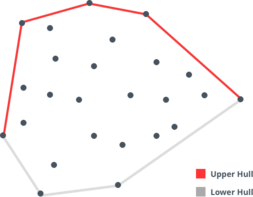C++ implementation of the above algorithm is as follows:

struct Point {
double x, y;
};
bool compare(Point a,Point b)
{
return a.x<b.x || (a.x==b.x && a.y<b.y);
}
//Returns positive value if B lies to the left of OA, negative if B lies to the right of OA, 0 if collinear
double cross(const Point &O, const Point &A, const Point &B)
{
return (A.x - O.x) * (B.y - O.y) - (A.y - O.y) * (B.x - O.x);
}
//Returns a list of points on the convex hull
vector<Point> convex_hull(vector<Point> P)
{
int n = P.size(), k = 0;
vector<Point> H(2*n);
sort(P.begin(), P.end(),compare);
// Build lower hull
for (int i = 0; i < n; ++i) {
while (k >= 2 && cross(H[k-2], H[k-1], P[i]) <= 0) k--;
H[k++] = P[i];
}

// Build upper hull
//i starts from n-2 because n-1 is the point which both hulls will have in common
//t=k+1 so that the upper hull has atleast two points to begin with
for (int i = n-2, t = k+1; i >= 0; i--) {
while (k >= t && cross(H[k-2], H[k-1], P[i]) <= 0) k--;
H[k++] = P[i];
}
//the last point of upper hull is same with the fist point of the lower hull
H.resize(k-1);
return H;
}



That was our convex hull using Andrew's algorithm, here we sorted using x coordinates for sweeping our line rightwards. This was using our line sweep technique.
Complexity of this algorithm is $O(N* log N)$ because of sorting. It may seem to be $O(N^2)$ because of while loop inside but this loop runs for overall $O(N)$ as we are deleting points in this loop and we have only N points , so it gives $O(N)$.

Now you have got some taste of this technique, now try solving the attached problem. Do explore this technique's application in some other problems.

Contributed by: Shubham Gupta
Уведомления

?